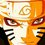# Olympiad Proof Problem - Day 2

This is the second proof problem I am posting. Try these problems and post your working. I will eventually post my solution to all of these notes, named "Olympiad Proof Problems", three days after I post them. So here is the problem.

Consider any ten distinct points $P_{1}$, $P_{2}$, $P_{3}$ $\ldots$ $P_{10}$ in the square $\mathcal{S}$ of unit side length. Prove that one can find a pair of points among those ten such that their distance is atmost $\dfrac{\sqrt{2}}{3}$ units.

Try more proof problems from this set Olympiad Proof ProblemsNote by Surya Prakash
5 years, 8 months ago

This discussion board is a place to discuss our Daily Challenges and the math and science related to those challenges. Explanations are more than just a solution — they should explain the steps and thinking strategies that you used to obtain the solution. Comments should further the discussion of math and science.

When posting on Brilliant:

• Use the emojis to react to an explanation, whether you're congratulating a job well done , or just really confused .
• Ask specific questions about the challenge or the steps in somebody's explanation. Well-posed questions can add a lot to the discussion, but posting "I don't understand!" doesn't help anyone.
• Try to contribute something new to the discussion, whether it is an extension, generalization or other idea related to the challenge.

MarkdownAppears as
*italics* or _italics_ italics
**bold** or __bold__ bold
- bulleted- list
• bulleted
• list
1. numbered2. list
1. numbered
2. list
Note: you must add a full line of space before and after lists for them to show up correctly
paragraph 1paragraph 2

paragraph 1

paragraph 2

[example link](https://brilliant.org)example link
> This is a quote
This is a quote
    # I indented these lines
# 4 spaces, and now they show
# up as a code block.

print "hello world"
# I indented these lines
# 4 spaces, and now they show
# up as a code block.

print "hello world"
MathAppears as
Remember to wrap math in $$ ... $$ or $ ... $ to ensure proper formatting.
2 \times 3 $2 \times 3$
2^{34} $2^{34}$
a_{i-1} $a_{i-1}$
\frac{2}{3} $\frac{2}{3}$
\sqrt{2} $\sqrt{2}$
\sum_{i=1}^3 $\sum_{i=1}^3$
\sin \theta $\sin \theta$
\boxed{123} $\boxed{123}$

Sort by:

Divide the unit square into $9$ small squares of side length $\frac {1}{3}$ . $10$ points are to be chosen within the unit square which means that there will be at least one small square with more than $1$ point. The maximum distance between any two points in such a square is when they are at diagonally opposite points of that square. By the Pythagorean theorem this distance is $\frac{\sqrt2}{3}$ .

- 5 years, 8 months ago

Nice solution!! (+1)

- 5 years, 8 months ago

It's just PHP followed by Pythagoras Theorem. Great solution BTW. +1

- 5 years, 8 months ago

Awesome solution!

- 5 years, 8 months ago

Here is a variant of the above question:

Consider a equilateral triangular dartboard of side 2ft. If you throw five darts with no misses, then show that at least two will be within one feet of each other.

###### Pakistan First Round 2015

- 5 years, 8 months ago

Once again, PHP followed by some simple maths.

Connect the medians of the triangle to create for equilateral triangles of side length 1ft. Now, at least 2 darts go into on of these triangles by PHP. The furthest distance 2 points can be separated in an equilateral triangle is the 2 vertices (pick any other 2 points and they are closer). This length is the side length, which is 1ft. Therefore, at least 2 darts are within 1ft of each other.

(Note: PHP stands for Pigeonhole Principle (or as I like to call it 'Penguin-hole Principle')

- 5 years, 8 months ago

I like to call it Pig-on-hole Principle.

- 5 years, 8 months ago

Easy PHP!

- 5 years, 8 months ago

Extremal Principle!!

- 5 years, 8 months ago

Actually I solved it in the paper with PHP..

- 5 years, 8 months ago

I solved it without paper using Penguin-hole Principle.

- 5 years, 8 months ago

WOW Sharky(-the genius) you win!

- 5 years, 8 months ago

ni shuo sha?

- 5 years, 8 months ago

- 5 years, 8 months ago

Nándào nǐ bù zhīdào yīngyǔ

- 5 years, 8 months ago